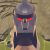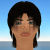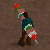## Welcome to the Second Life Forums Archive

These forums are CLOSED. Please visit the new forums HERE

# Bitfield manipulation functions

Huns Valen
Don't PM me here.Join date: 3 May 2003
Posts: 2,749
04-03-2004 02:03
I realized that having huge arrays of ints to store TRUE/FALSE wasn't memory efficient, so I decided to pack the info into bitfields instead. Please note that the bits are set in RIGHT TO LEFT order, counting from zero. Setting bit zero gives you the number '00000001', setting bit seven gives you the number '10000000'.

CODE
`// Sets a bit in an integer bitfield and returns the bitfieldinteger setBit(integer x, integer bit) {    return x | ((integer)llPow(2, (float)bit));}// Clears a bit in an integer bitfield and returns the bitfieldinteger clearBit(integer x, integer bit) {    return x & (~(integer)llPow(2, (float)bit));}// Reads a bit in an integer bitfield and returns the bitinteger getBit(integer x, integer bit) {    if(x & (integer)llPow(2, bit))        return TRUE;    else        return FALSE;}default {    state_entry() {        // test: 106 (binary 01101010) and 42 (binary 00101010)        integer x;        x = setBit(x, 1);        x = setBit(x, 3);        x = setBit(x, 5);        x = setBit(x, 6);        llSay(0, (string)x);        x = clearBit(x, 6);        llSay(0, (string)x);        integer i;        integer j = 8;        for(i=0; i<j; i++)            llSay(0, (string)x+" bit 2^"+(string)i+"="+(string)getBit(x, i));            }}`

When compiled, this is the output of the test:
Object: 106
Object: 42
Object: 42 bit 2^0=0
Object: 42 bit 2^1=1
Object: 42 bit 2^2=0
Object: 42 bit 2^3=1
Object: 42 bit 2^4=0
Object: 42 bit 2^5=1
Object: 42 bit 2^6=0
Object: 42 bit 2^7=0
_____________________
Huns Valen
Don't PM me here.Join date: 3 May 2003
Posts: 2,749
int2bin
04-03-2004 22:48
Also:

// Return a string containing a binary representation of an int
string int2Bin(integer int, integer places) {
integer i;
string bin;
for(i=places - 1; i>-1; i--)
bin += (string)getBit(int, i);
return bin;
}

This lets you print out the binary value of an int. For example, int2Bin(256, 9) would return 100000000.
_____________________
wizzie Baldwin
Registered UserJoin date: 23 May 2004
Posts: 52
another approach...
06-11-2004 20:52
hi,
nice work.

i'm very new to LSL about 45 min. or so.

I don't know if this is relevant to this discussion, but here are some more tricks i used to use a long time ago (when it was very necessary) to keep mem to a minimum.

Also since i'm new and have not read all of the doc's yet
some of the stuff may not apply. Sorry if it doesn't

if this is something u already know then i apologize for wasting any time.

variable declarations (if LSL allows) for bools, shorts can conserve mem.

It appears the languge is like C. If structures are allowed there was the ability in C to do bitwize storage there and also with unions if i remember correctly.

Also another one of my favorites was using binray masks
(i'm not sure if defines are allowed but it's the approach i'm trying to explain)

#define A 0x1;
#define B 0x2;
#define C 0x4;
#define D 0x8;
...
#define i 0x100; // 256

you can assign any number of bits to be relevant for any given condition
and it's easy to check a condition

if the variable in question has bits (A&E&F) set or any other combination do something. It allows for n! choices to number of bits u choose to use in ways you can combine them for your own meaning.

regards,
wizzie
gene Poole
"Foolish humans!"Join date: 16 Jun 2004
Posts: 324
03-18-2005 22:34
Not that it's necessarily more efficient, but you might consider using shifts instead of calling llPow() -- they are in fact, to me, more readable (WRT the meaning of the code).
Huns Valen
Don't PM me here.Join date: 3 May 2003
Posts: 2,749
03-21-2005 01:46
From: someone
Not that it's necessarily more efficient, but you might consider using shifts instead of calling llPow() -- they are in fact, to me, more readable (WRT the meaning of the code).
This thread predates the bitshifting functions in LSL. It would probably be better to use >> and <<, now that we have them.
_____________________